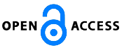Isaac Scientific Publishing### Improved Estimator of Measure for Marginal Homogeneity using Marginal Odds in Square Contingency Tables

Download PDF (360.1 KB) PP. 71 - 77 Pub. Date: June 13, 2017

### Author(s)

• Kiyotaka Iki*
Department of Information Sciences, Faculty of Science and Technology, Tokyo University of Science, Noda City, Chiba, Japan

Department of Information Sciences, Faculty of Science and Technology, Tokyo University of Science, Noda City, Chiba, Japan

### Abstract

For square contingency tables, Iki, Tahata and Tomizawa (2011) considered the measure to represent the degree of departure from the marginal homogeneity model. Using the first-order term in the Taylor series expansion, the estimated measure with the cell probabilities replaced by the corresponding sample proportions is an approximately unbiased estimator when the sample size is large. The present paper proposes the improved approximate unbiased estimator of the measure which is obtained by using the second-order term in the Taylor series expansion. Also, it shows that the improved estimator approaches to the true measure faster than the original estimator as the sample size becomes larger by the simulation studies.

### Keywords

Estimation, marginal homogeneity, marginal odds, square contingency table, taylor series expansion, unbiased estimator.

### References

 Agresti, A. (2013). Categorical Data Analysis, 3rd ed. New Jersey: Wiley.

 Bishop, Y. M. M., Fienberg, S. E., and Holland, P. W. (1975). Discrete Multivariate Analysis: Theory and Practice. Cambridge: The MIT Press.

 Iki, K., Tahata, K. and Tomizawa, S. (2011). Measure of departure from marginal homogeneity using marginal odds for multi-way tables with ordered categories. Journal of Applied Statistics, Vol. 39, pp. 279-295.

 Patil, G. P. and Taillie, C. (1982). Diversity as a concept and its measurement. Journal of the American Statistical Association, Vol. 77, pp. 548-561.

 Read, T. R. C. and Cressie, N. (1988). Goodness-of-Fit Statistics for Discrete Multivariate Data. New York: Springer-Verlag.

 Stuart, A. (1955). A test for homogeneity of the marginal distributions in a two-way classification. Biometrika, Vol. 42, pp. 412-416.

 Tahata, K., Tanaka, H. and Tomizawa, S. (2014). Refined estimators of measures for marginal homogeneity in square contingency tables. International Journal of Pure and Applied Mathematics, Vol. 90, pp. 501-513.

 Tomizawa, S. and Makii, T. (2001). Generalized measures of departure from marginal homogeneity for contingency tables with nominal categories. Journal of Statistical Research, Vol. 35, pp. 1-24.

 Tomizawa, S., Miyamoto, N. and Ashihara, N. (2003). Measure of departure from marginal homogeneity for square contingency tables having ordered categories. Behaviormetrika, Vol. 30, pp. 173-193.